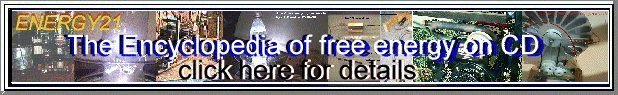## Supplemental information on Paschen's Law, equations, breakdown in gases.

This is really rough, still....

Paschen's Law reflects the Townsend breakdown mechanism in gases, that is, a cascading of secondary electrons emitted by collisions in the gap. The significant parameter is pd, the product of the gap distance and the pressure. Typically, the Townsend mechanism (and by extension Paschen's law) apply at pd products less than 1000 torr cm, or gaps around a centimeter at one atmosphere. Furthermore, some modifications are necessary for highly electronegative gases because they recombine the secondary electrons very quickly.

In general, an equation for breakdown is derived, and suitable parameters chosen by fitting to empirical data.

Here are three equations:

Breakdown voltage:
Vbreakdown = B * p * d / (C + ln( p * d))

Breakdown field strength:
Ebreakdown = p * ( B / ( C + ln ( p * d)))

where:
C = A / ln ( 1 + 1 / gamma)

where:
gamma is the (poorly known) secondary ionization coefficient.

For air:
A = 15 cm-1Torr -1
B = 365 Vcm-1 Torr-1
and gamma = 10-2
so
C = 1.18

above data taken from Bazelyan, p.32

Minimum sparking potential for various gases

GasVs min
(V)
pd at Vs min
(torr cm)
Air3270.567
Ar1370.9
H22731.15
He1564.0
CO24200.51
N22510.67
N2O4180.5
O24500.7
SO24570.33
H2S4140.6

data from Naidu, p.27

Note that the sparking voltage us affected by the electrode material, with cathodes of Barium and Magnesium having higher voltages than Alumninum, for example.

#### Temperature dependence

Paschen's law ( V = f(pd)) should really be stated as V = f( Nd) where N is the density of gas molecules, which is, of course, affected by the temperature as well as the pressure of the gas ( n/V = p/RT). An empirical formula for air is:

x = 293 * p * d / (760 * T)
Vbreakdown = 24.22 * x + 6.08 * SQRT(x)

#### Gamma - Townsend's secondary ionization coefficient

Gamma is the net (!) number of secondary electrons produced per incident positive ion, photon, excited or metastable particle. It is a function of gas pressure and E/p. Electronegative gases (SF6, Freon, oxygen, CO2) reattach the electrons very quickly, so they have low gammas.

For nitrogen, gamma ranges between 10-3 and 10-2 for E/p of 100-700 V cm-1 torr-1. Insulating gases like SF6 or Freon have gammas of 10-4 or even less.

A further reference for information would be Cobine.

Copyright 1998, Jim Lux / paschen2.htm / 28 March 1998 / Back to HV Home / Back to home page / Mail to Jim